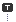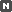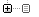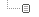Every unexpected event is a path to learning for you.

### 카테고리분류 전체보기 (2342)Unity3D (577)Programming (473)Unreal (4)Gamebryo (56)Tip & Tech (187)협업 (34)3DS Max (3)Game (12)Utility (116)Etc (92)Portfolio (19)Subject (90)iOS,OSX (38)Android (13)Linux (5)잉여 프로젝트 (2)게임이야기 (1)Memories (20)Interest (37)Thinking (36)한글 (26)PaperCraft (5)Animation (408)Wallpaper (2)재테크 (19)Exercise (3)나만의 맛집 (2)냥이 (9)육아 (13)
Total1,356,270
Today38
Yesterday269### 달력

« » 2020.1
1 2 3 4
5 6 7 8 9 10 11
12 13 14 15 16 17 18
19 20 21 22 23 24 25
26 27 28 29 30 31

## C# Win32 messaging with SendMessage and WM_COPYDATA

Programming/C# / 2012. 5. 8. 16:47

I had a real pain recently where I wanted to control one windows app from another. I found some useful stuff on the net, but nothing that gave an end to end solution. So here’s what I came up with.

Firstly I’ll explain why this is useful. SendMessage is part of the Win32 API, and is used to send messages from one application to another. There are a set of predefined properties that the message can relate to, and these can be used to send messages to existing applications to perform all sorts of useful functions such as changing the font in notepad, or bringing a window to the fore. For more information of the wider use of the SendMessage function, have a look at:

http://www.autohotkey.com/docs/commands/PostMessage.htm

http://msdn.microsoft.com/en-us/library/ms644950(VS.85).aspx

The main use that I’m interested in is passing a specific instruction (via a string) from one app that I’ve written, to another one that I’ve written. This way I can effectively remote control one app from another (particularly useful if you want your main application to open a pop-up, and you don’t want to worry about the pop-up’s performance affecting the main application). Let’s now have a quick look at the SendMessage function:

SendMessage(int hWnd, int Msg, int wParam, int lParam)

hWnd – This is the window instance id of the application you want to send a message to. This id is retrieved using the FindWindow function

Msg – This is the type of message you want to send

wParam – Message specific data you pass in

wParam – Message specific data you pass in

Also used is the FindWindow function. This is to get the relevant window id:

FindWindow(String lpClassName, String lpWindowName)

lpClassName -The name of the class you want

lpWindowName – The name of the window that you want

To send a message that is a string, you need to use the WM_DATACOPY message property. The hard part is that you cannot just send the string as a parameter across. You need to send a pointer to the memory address of the string. If you just want to send an integer as a message you can use the WM_USER message property and send it as a value without a problem.

Below now is a brief listing of my MessageHelper.cs class, for the whole class file see:

http://craigcook.co.uk/samples/MessageHelper.cs.txt

 `01` `using` `System;`
 `02` `using` `System.Collections.Generic;`
 `03` `using` `System.Linq;`
 `04` `using` `System.Text;`
 `05` `using` `System.Runtime.Serialization.Formatters.Binary;`
 `06` `using` `System.Runtime.InteropServices;`
 `07` `using` `System.Diagnostics;`
 `08`
 `09` `public` `class` `MessageHelper`
 `10` `{`
 `11` `[DllImport(``"User32.dll"``)]`
 `12` `private` `static` `extern` `int` `RegisterWindowMessage(``string` `lpString);`
 `13`
 `14` `[DllImport(``"User32.dll"``, EntryPoint = ``"FindWindow"``)]`
 `15` `public` `static` `extern` `Int32 FindWindow(String lpClassName, String lpWindowName);`
 `16`
 `17` `//For use with WM_COPYDATA and COPYDATASTRUCT`
 `18` `[DllImport(``"User32.dll"``, EntryPoint = ``"SendMessage"``)]`
 `19` `public` `static` `extern` `int` `SendMessage(``int` `hWnd, ``int` `Msg, ``int` `wParam, ``ref``COPYDATASTRUCT lParam);`
 `20`
 `21` `//For use with WM_COPYDATA and COPYDATASTRUCT`
 `22` `[DllImport(``"User32.dll"``, EntryPoint = ``"PostMessage"``)]`
 `23` `public` `static` `extern` `int` `PostMessage(``int` `hWnd, ``int` `Msg, ``int` `wParam, ``ref``COPYDATASTRUCT lParam);`
 `24`
 `25` `[DllImport(``"User32.dll"``, EntryPoint = ``"SendMessage"``)]`
 `26` `public` `static` `extern` `int` `SendMessage(``int` `hWnd, ``int` `Msg, ``int` `wParam, ``int``lParam);`
 `27`
 `28` `[DllImport(``"User32.dll"``, EntryPoint = ``"PostMessage"``)]`
 `29` `public` `static` `extern` `int` `PostMessage(``int` `hWnd, ``int` `Msg, ``int` `wParam, ``int``lParam);`
 `30`
 `31` `[DllImport(``"User32.dll"``, EntryPoint = ``"SetForegroundWindow"``)]`
 `32` `public` `static` `extern` `bool` `SetForegroundWindow(``int` `hWnd);`
 `33`
 `34` `public` `const` `int` `WM_USER = 0x400;`
 `35` `public` `const` `int` `WM_COPYDATA = 0x4A;`
 `36`
 `37` `//Used for WM_COPYDATA for string messages`
 `38` `public` `struct` `COPYDATASTRUCT`
 `39` `{`
 `40` `     public` `IntPtr dwData;`
 `41` `     public` `int` `cbData;`
 `42` `     [MarshalAs(UnmanagedType.LPStr)]`
 `43` `     public` `string` `lpData;`
 `44` `}`
 `45`
 `46` `public` `bool` `bringAppToFront(``int` `hWnd)`
 `47` `{`
 `48` `     return` `SetForegroundWindow(hWnd);`
 `49` `}`
 `50`
 `51` `public` `int` `sendWindowsStringMessage(``int` `hWnd, ``int` `wParam, ``string` `msg)`
 `52` `{`
 `53` `    int` `result = 0;`
 `54`
 `55` `     if` `(hWnd != 0)`
 `56` `     {`
 `57` ```            byte[] sarr = System.Text.Encoding.Default.GetBytes(msg);```
 `58` ```            int len = sarr.Length;```
 `59` ```            COPYDATASTRUCT cds;```
 `60` ```            cds.dwData = (IntPtr)100;```
 `61` ```            cds.lpData = msg;```
 `62` ```            cds.cbData = len + 1;```
 `63` ```            result = SendMessage(hWnd, WM_COPYDATA, wParam, ref cds);```
 `64` `     }`
 `65`
 `66` `     return` `result;`
 `67` `}`
 `68`
 `69` `public` `int` `sendWindowsMessage(`